ChemTalk

# Coulomb’s Law## Core Concepts

This tutorial will teach you about Coulomb’s law and its application in calculating the force between two electrically charged particles.

## Topics Covered in Other Articles

Coulomb’s law states that like charges repel while opposite charges attract with a force that is directly proportional to the product of their charges. Further, this force is inversely proportional to the square of the distance between them. In 1785, French physicist Charles-Augustin de Coulomb showed that opposite charges attract according to this inverse square law: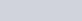## Breaking down the equation

To begin, we’ll look at two charged bodies 1 and 2 which are separated by a distance of r meters from each other.

According to Coulomb’s law, the electrostatic force between the two point charges is directly proportional to the product of the charges on them.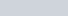The law also states that the electrostatic force is inversely proportional to the square of the distance between the two point charges.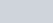After combining these two relationships together we get: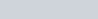When Coulomb’s constant, a proportionality constant that depends on the medium in which the point charges are placed, is introduced, this equation transforms into Coulomb’s law. Written below is Coulomb’s constant, denoted as ke.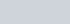The constant ε0 is known as the electric constant and is equal to 8.85 x 10-12 farads per meter (F/m). In SI units, Coulomb’s constant has the value of ke= 8.99 x 109 Nm2C-2. With this knowledge, we now understand that we can express Coulomb’s law in a few different ways. I will share these below:If the two point charges are the same, the force between the particles is repulsive and will lead to a positive result with Coulomb’s law. Contrarily, if the two point charges are opposite, the ore between the particles is attractive and will lead to a negative result with Coulomb’s law.

### Limitations

Coulomb’s law does have some limitations that we will note.

• First, Coulomb’s law is only applicable when the point charges are at rest. This is because bringing the two charges together will change the distribution of their charges.
• Secondly, if the shape of the point charges is irregular, Coulomb’s law cannot be applied. This is because it is difficult to determine the distance between the irregularly shaped particles.
• Thirdly, Coulomb’s law cannot be used to calculate the charges on big planets.

## Coulomb’s Law Experiment

Charles Coulomb had a degree in engineering and was a trained army engineer. After working on many engineering projects, he started to focus his attention on physics in the 1780s. He was interested in measuring the electrostatic force between charged objects. In order to measure these small forces, he used a torsion balance.

In Coulomb’s experiment, he had a needle that held a small electrically charged pith ball on one end and a counterweight on the other. Coulomb suspended the needle on a fine fiber and balanced it so it could rotate in a horizontal plane. He measured the force needed to twist the needle at any given angle using the calibrated torsion balance. When the two pith balls had similar charges, Coulomb determined the repulsive force between them as a function.

Coulomb ran into some difficulties when the two pith balls had opposite charges. Ultimately, he decided to use a different method to achieve his results. He suspended a needle with a small, charged plate and made it oscillate in a narrow arc. Then, he placed the opposite charge on the surface of a hollow sphere. Coulomb measured the oscillation period at different distances from the sphere and related the period to the force between the charges.

## The Coulomb Potential

The Coulomb potential describes two types of situations. First, it can explain continuous states where energy is greater than zero (E>0) like when electrons and protons scatter. Second, it also helps us understand situations where electrons hold close to protons in specific energy levels (discrete bound states). This becomes relevant when explaining the structure of hydrogen atoms.

The equation for the Coulomb potential (also called the electric potential) is: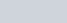Where V is the Coulomb potential (electric potential), ke is Coulomb’s constant, Q is the point charge, and r is the distance from the charge point where the potential is being measured.

## Coulomb’s Law Practice Problems

1. Calculate the electrostatic force between two charges, 9 x 10-8 C and 4 x 10-7 C. There is a separation of 25 cm between these charges in free space.
2. Coulomb’s law is applicable for ___.
• Charges that are in an irregular shape.
• Point charges at rest.
• Charges of big planets.
3. The value of ke in Coulomb’s law depends on ___.
• The medium between the two charges.
• The distance between the two charges.
• The magnitude of the charges.

## Coulomb’s Law Practice Problem Solutions

Problem 1Problem 2

Point charges at rest.

Problem 3

The medium between the two charges.